The Miscellaneous Right Triangles ClipArt gallery includes 30 illustrations including the special triangles known as the 30-60-90 and the 45-45-90. There are also images of leaning ladders, buildings, and trees that can be used with application problems involving right triangle trigonometry and use of the Pythagorean theorem.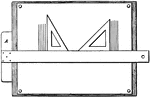### Drawing Board and Triangles

Drawing board with t-square and two triangles.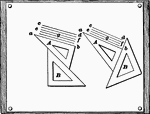### Drawing Parallel Lines

Using a drawing board and pairs of triangles to draw parallel lines.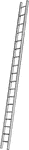Illustration of a ladder that is not perpendicular to the ground. If it is set on the ground and leaned…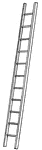Illustration of a ladder that is not perpendicular to the ground. If it is set on the ground and leaned…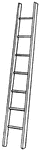Illustration of a ladder that is not perpendicular to the ground. If it is set on the ground and leaned…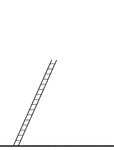Illustration of a ladder that is not perpendicular to the ground. If it is set on the ground and leaned…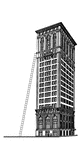### Ladder Leaning Against a Building

Illustration of a ladder leaning against the side of a building (wall) to form a right triangle .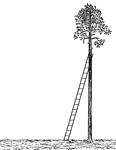### Ladder Leaning Against a Tree

Illustration of a ladder leaning against a palm tree, that is perpendicular to the ground, to form a…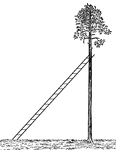### Ladder Leaning Against a Tree

Illustration of a ladder leaning against a palm tree, that is perpendicular to the ground, to form a…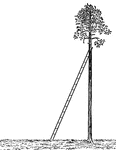### Ladder Leaning Against a Tree

Illustration of a ladder leaning against a palm tree, that is perpendicular to the ground, to form a…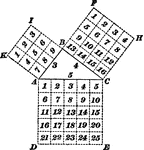### Right Triangle

"In any right triangle, the square described on the hypotenuse is equal to the sum of the squares described…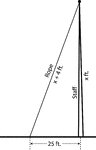### Right Triangle Formed by Flagpole (x feet high) and Ground With Rope (x+4 feet)

An illustration of a right triangle made up of a flagpole, rope, and the ground. Illustration could…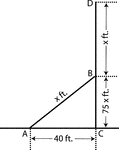### Right Triangle With Sides x, 75, and 40

An illustration of a right triangle with sides, 40, 75, and x. Additional height of x is added to 75.…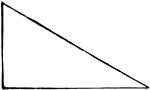### Right-Angled

A right-angled triangle is any triangle having one right angle. The side opposite the right angle is…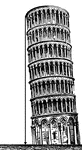### Leaning Tower

Illustration of a leaning tower with a perpendicular drawn from the top of the tower to the ground to…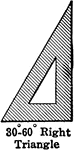### 30-60-90 Right Triangle

30-60-90 right triangle.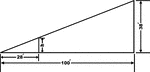### Inclined Plane Triangle

Inclined plane using right triangle and proportions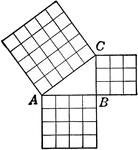### Pythagorean Theorem Triangle

Right triangle with squares that can be used to illustrate the Pythagorean Theorem.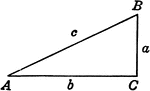### Right Triangle ABC

Right triangle with sides a, b, c and angles A, B, C labeled.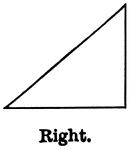### Right Triangle

Illustration showing a right triangle (one that has one right angle).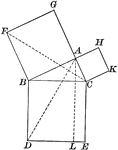### Right Triangle

Illustration of a right triangle used to show the Pythagorean Theorem (the square of the hypotenuse…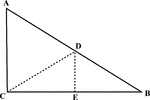### Right Triangle With Midpoint of Hypotenuse Drawn

Illustration of a right triangle with the midpoint of the hypotenuse drawn.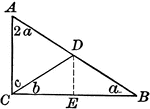### Right Triangle With One Angle Double the Other

Illustration of a right triangle with one angle the double of the other.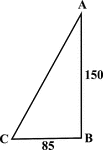### Right Triangle With Sides 85 and 150

Illustration of a right triangle with legs labeled 150 and 85. This could be used to find the hypotenuse…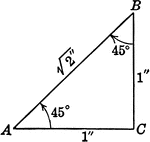### Special Right Triangle with Angles 45, 45, 90 degrees

Special right triangle with angles 45 degrees, 45 degrees, and 90 degrees with side measures/relationships…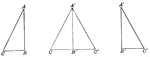### Equal Right Triangles by Hypotenuse Leg

Illustration showing two equal right triangles. This can be used to show that two right triangles are…